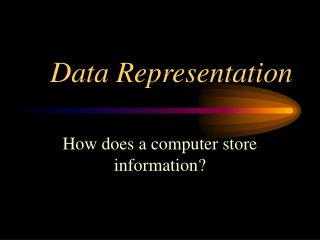DownloadDownload PresentationData Representation

# Data Representation

Télécharger la présentation## Data Representation

- - - - - - - - - - - - - - - - - - - - - - - - - - - E N D - - - - - - - - - - - - - - - - - - - - - - - - - - -
##### Presentation Transcript

1. Data Representation How does a computer store information?

2. Pulses of electricity • A pulse is 1 • no pulse is 0

3. Pulses come in groups of 8known as a byte 1 0 1 1 1 0 1 1 Each 0 and 1 is known as a bit

4. a number a character a program instruction eg the number ‘24’ eg the letter ‘A’ eg the instruction ‘Add’ The pattern of 0’s and 1’s may be:-

5. Numbers and Humans • We use a number system called decimal • It uses ten digits (0 1 2 3 4 5 6 7 8 9) • Good for humans with 10 fingers!!

6. Numbers and Computers • Computers use a number system called binary. • It uses two digits (0 and 1) • Good for machines with a pulse (1) or no pulse (0)

7. Remember your earliest sums in primary school ‘Hundreds, Tens & Units’ H T U 100 10 1 (100 x 6) = 600 (10 x 5) = 50 (1 x 3) = 3 Total = 65310 Decimal Numbers (Base 10) Let’s look at:- 100 10 1 6 5 3 which becomes:- Notice how the numbers (place values) multiply by 10 as we move from right to left

8. Instead of : ‘Hundreds, Tens & Units’ we have: ‘Eights, Fours, Twos and Units’ 8 4 2 1 (8 x 1) = 8 (4 x 1) = 4 (2 x 0) = 0 (1 x 1) = 1 Total = 1310 Binary Numbers (Base 2) Let’s look at:- 8 4 2 1 1 1 0 1 which becomes:- Notice how the numbers (place values) multiply by 2 as we move from right to left

9. Changing Binary to Decimal